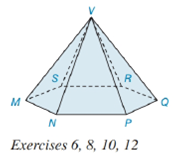Chapter 9.2, Problem 10EElementary Geometry For College St...

7th Edition
Alexander + 2 others
ISBN: 9781337614085

Solutions

Chapter
SectionElementary Geometry For College St...

7th Edition
Alexander + 2 others
ISBN: 9781337614085
Textbook Problem

Suppose that the base of the hexagonal pyramid in Exercise 6 has an area of 41.6 c m 2 and that of each lateral face has an area of 20 c m 2 . Find the total (surface) area of the pyramid.To determine

To find:

The total surface area of the given pyramid.

Explanation

Given:

The figure given below

and base of the hexagonal pyramid has an area of 41.6cm2 and each lateral face has an area of 20cm2.

Formula Used:

A pyramid is made by connecting a base to an apex or vertex. The base is flat with straight edges, no curves, hence, a polygon and all other faces are triangles.

A regular pyramid is a pyramid whose base is a regular polygon and whose lateral edges are all congruent.

The lateral faces are given by the triangular faces of the pyramid.

The total surface area T of a pyramid with lateral area A and base area B is given by

T=L+B.

The area of a square B of side a is given by

B=a2.

Calculation:

From the given figure

The apex is directly above the center of the base. Hence it is a right Pyramid and the lateral edges are congruent.

The lateral faces are congruent. Thus, a regular pyramid.

The number of lateral faces is given by the triangular faces of the pyramid i

Still sussing out bartleby?

Check out a sample textbook solution.

See a sample solution

The Solution to Your Study Problems

Bartleby provides explanations to thousands of textbook problems written by our experts, many with advanced degrees!

Get Started

Solve the equations in Exercises 112 for x (mentally, if possible). 4x5=8

Finite Mathematics and Applied Calculus (MindTap Course List)

Rewrite by completing the square. (a) x2 + x + 1 (b) 2x2 12x + 11

Single Variable Calculus: Early Transcendentals, Volume I

Find the limit of f(x) as x approaches 0. f(x)={ x2+1,x02x+1,x0

Calculus: An Applied Approach (MindTap Course List)

How many different batting orders can be formed for a nine-member baseball team?

Finite Mathematics for the Managerial, Life, and Social Sciences